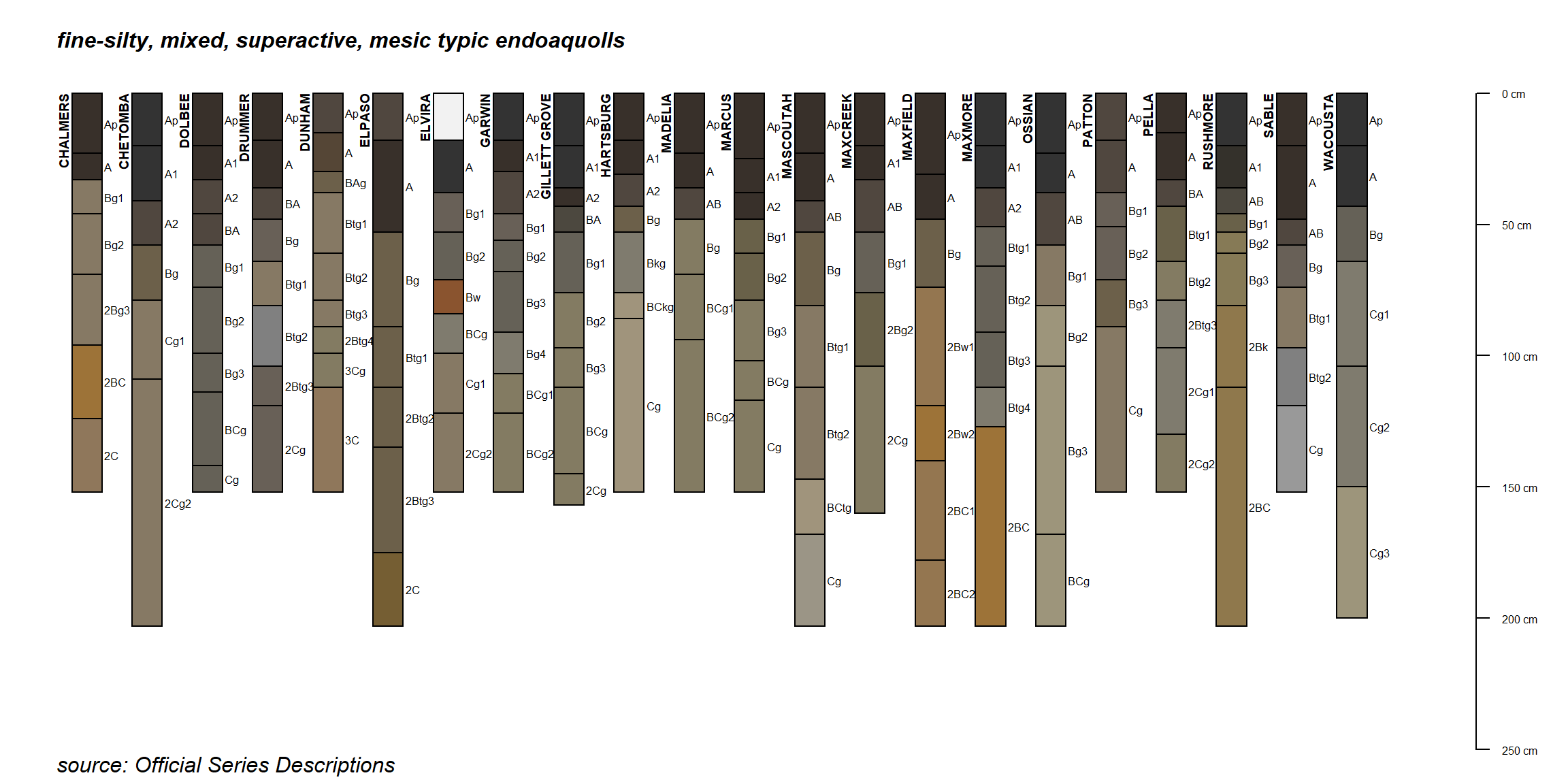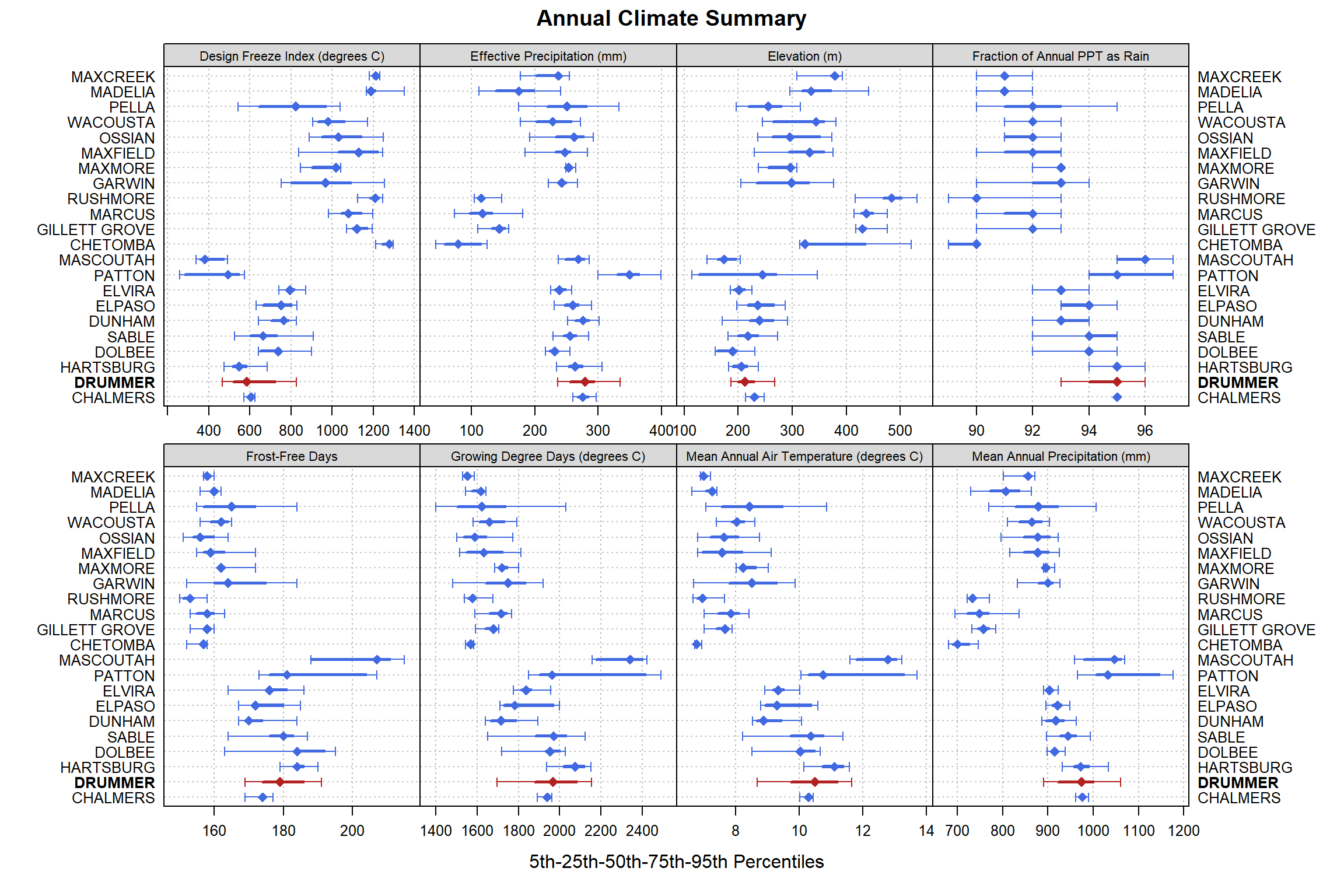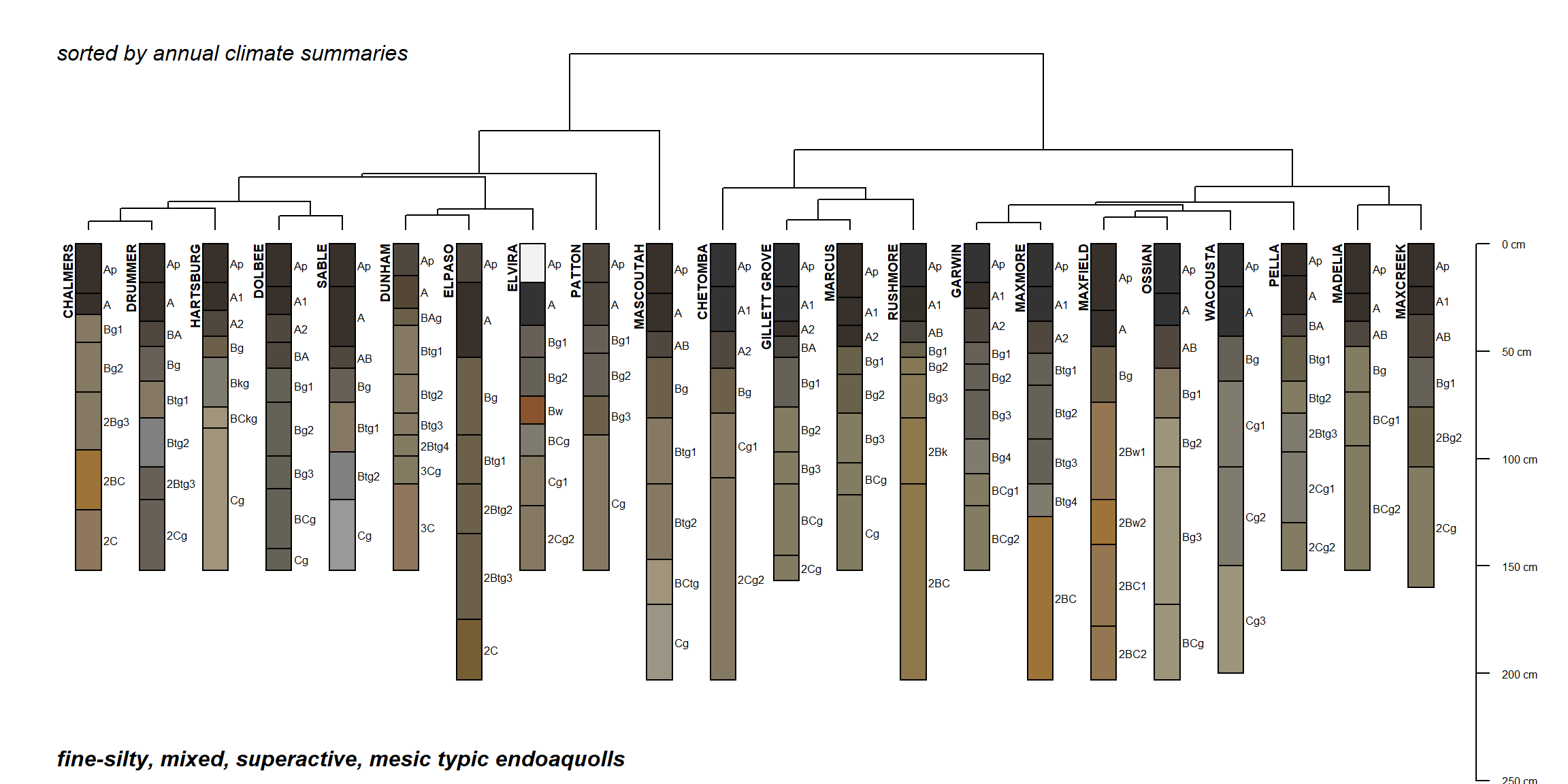## Prologue

The soil series concept is a practical grouping of soil/landscape patches that require a unique set of management / conservation practices. The revision of old, establishment of new, and correlation among series requires data. A consistent set of data promotes consistency in the definition and use of series concepts. There are over 24,000 soil series in the US. Do we need them all? Do we need more? Which ones need work? How do we keep track of data and specific exemplars used to define the series? These are all questions that USDA-NRCS soil scientists struggle with. A more clearly defined soil series concept directly impacts our ability to communicate a “package” of soil / landscape parameters to other scientists and, most importantly, to those who work with the soil. Soil survey is much more than making maps and little sketches. It is a systematic catalog of our best attempt at figuring out how soils vary on the landscape, so that we can make the best use of the soil resource. Why this matters is not, but should be obvious: food, fiber, fuel.

## Practical Matters

This is rough outline for evalutating potential differences between soil series within the same family, e.g. competing series. For this example, start the process by loading data within the same family as the Drummer series; fine-silty, mixed, superactive, mesic typic endoaquolls.

Why would a soil scientist do this?

• reviewing the criteria for competing soil series is problematic when OSDs haven’t been updated
• this process should be less subjective and as quantitative as possible
• there is usually more to the story than a single “difference” between series

## Setup

Load relevant packages and define some helper functions.

``````# you will need the latest version of soilDB
library(soilDB)
library(aqp)
library(sharpshootR)
library(latticeExtra)
library(RColorBrewer)
library(reshape2)
library(cluster)
library(ape)
library(scales)
library(maps)``````

## Get Data

Get extended summaries for the Drummer soil series. As of `soilDB` 2.3 this includes a list of “competing” soil series.

``````soil <- 'DRUMMER'
s <- fetchOSD(soil, extended = TRUE)``````

Get extended summaries for Drummer and competing series.

``````# get competing series OSD data
spc <- fetchOSD(c(soil, s\$competing\$competing))

# this will only work for established series, e.g. those that have been "mapped" somewhere
idx <- which(spc\$series_status == 'established')
spc <- spc[idx, ]

# save family taxa and set of series names for later
fm.name <- unique(na.omit(spc\$family))
s.names <- unique(site(spc)\$id)``````

### First Look

Plot sketches of the soils in this family, data are from OSDs via SoilWeb.

``````# plot
par(mar=c(0.25,0,1,1))
plot(spc)
mtext(fm.name, side = 3, at = 0.5, adj = 0, line = -1, font=4)
mtext('source: Official Series Descriptions', side = 1, at = 0.5, adj = 0, line = -1, font=3, cex=1)``````## Climate Summaries

Compare annual climate summaries using divisive hierarchical clustering of median values.

``````# get OSD + extended summaries for all competing series
s.competing.data <- fetchOSD(s.names, extended = TRUE)``````

Evaluate individual climate variables using select percentiles. Soil series labels are sorted according to divisive hierarchical clustering. The two main groupings are clearly visible in those climate varibles linked to mean annual air temperature.

``````# control color like this
trellis.par.set(plot.line=list(col='RoyalBlue'))

# control centers symbol and size here
res <- vizAnnualClimate(s.competing.data\$climate.annual, s=soil, IQR.cex = 1.1, cex=1.1, pch=18)

print(res\$fig)``````Vizualize pair-wise distances using a dendrogram and profile sketches. What do the two main groupings tell us about this family?

``````par(mar=c(0,0,1,1))
plotProfileDendrogram(s.competing.data\$SPC, clust = res\$clust, scaling.factor = 0.075, width = 0.2, y.offset = 0.5)
mtext(fm.name, side = 1, at = 0.5, adj = 0, line = -1.5, font=4)
mtext('sorted by annual climate summaries', side = 3, at = 0.5, adj = 0, line = -1.5, font=3)``````### Monthly Climate Summaries

``````library(ggplot2)
# reasonable colors for a couple of groups
cols <- brewer.pal(9, 'Set1')
cols <- cols[c(1:5,7,9)]

idx <- which(s.competing.data\$climate.monthly\$series %in% c('DRUMMER', 'MARCUS', 'PATTON', 'MAXCREEK') & s.competing.data\$climate.monthly\$variable == 'Precipitation (mm)')
s.sub <- s.competing.data\$climate.monthly[idx, ]

ggplot(s.sub, aes(x = month, group=series)) +
geom_ribbon(aes(ymin = q25, ymax = q75, fill=series)) +
geom_line(aes(month, q25)) +
geom_line(aes(month, q75)) +
geom_abline(intercept=0, slope = 0, lty=2) +
xlab('') + ylab('mm') +
ggtitle('Monthly IQR') +
scale_fill_manual(values=alpha(cols, 0.75)) +
facet_wrap(vars(variable), scales = 'free_y') +
theme_bw()``````